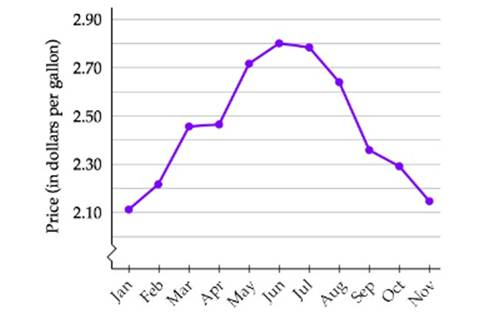Chapter 2.1, Problem 51ESMathematical Excursions (MindTap C...

4th Edition
Richard N. Aufmann + 3 others
ISBN: 9781305965584

Solutions

Chapter
SectionMathematical Excursions (MindTap C...

4th Edition
Richard N. Aufmann + 3 others
ISBN: 9781305965584
Textbook Problem

Gasoline Prices The following broken line graph shows the average U.S. monthly regular gasoline prices for the months from January to November of 2015.Use the information in the above graph and the roster method to represent each of the following sets.a. The set of months in which the price of gasoline was greater than $2.50 per gallon.b. The set of months in which the price of gasoline was greater than$2.30 but less than $2.60 per gallon.c. The set of months in which the price of gasoline was less than S2.20 per gallon. a. To determine To determine the sets using roster method. Explanation Given: The set of months in which the price of gasoline was greater than$2.50pergallon

The set of months in which the price of gasoline was greater than \$2

b.

To determine

To determine the sets using roster method.

c.

To determine

To determine the sets using roster method.

Still sussing out bartleby?

Check out a sample textbook solution.

See a sample solution

The Solution to Your Study Problems

Bartleby provides explanations to thousands of textbook problems written by our experts, many with advanced degrees!

Get Started

Determine the infinite limit. limx0+(1xlnx)

Single Variable Calculus: Early Transcendentals, Volume I

Explain why the formula for sample variance is different from the formula for population variance.

Essentials of Statistics for The Behavioral Sciences (MindTap Course List)

True or False:

Study Guide for Stewart's Single Variable Calculus: Early Transcendentals, 8th

True or False: converges.

Study Guide for Stewart's Multivariable Calculus, 8th